Important Definitions & Formulas: Triangles

# Important Definitions & Formulas: Triangles - Notes | Study Mathematics (Maths) Class 10 - Class 10

 Table of contentsSimilar PolygonsBasic Proportionality Theorem (or Thales Theorem)Converse of Basic Proportionality TheoremCriteria for Similarity of Triangles:Areas of Similar TrianglesPythagoras Theorem1 Crore+ students have signed up on EduRev. Have you?

Similar Polygons

Two polygons with the same number of sides are said to be similar, if
(i) their corresponding angles are equal and
(ii) their corresponding sides are in proportion (or are in the same ratio).

Basic Proportionality Theorem (or Thales Theorem)

If a line is drawn parallel to one side of a triangle intersecting the other two sides, then it divides the two sides in the same ratio.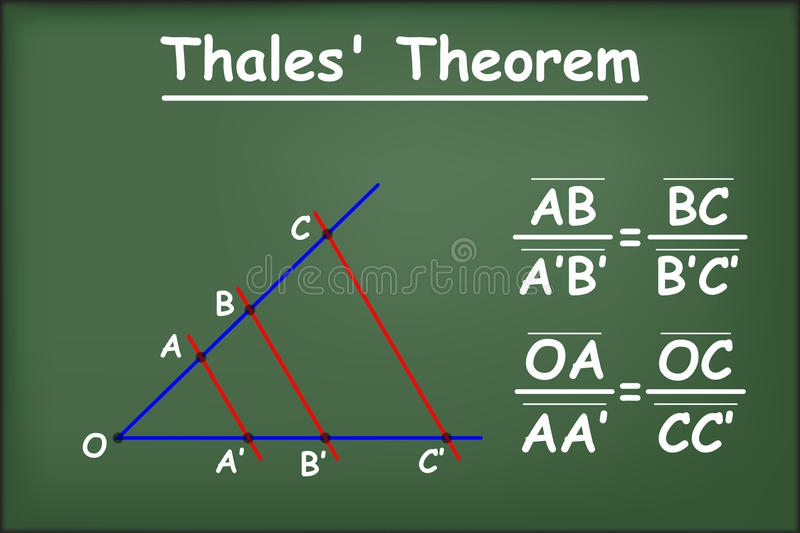Thales Theorem

OrIf a line is drawn parallel to one side of a triangle, to intersect the other two sides in distinct points, the other two sides are divided in the same ratio i.e., If in ΔABC, l ||BC, intersecting in D and E, then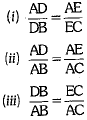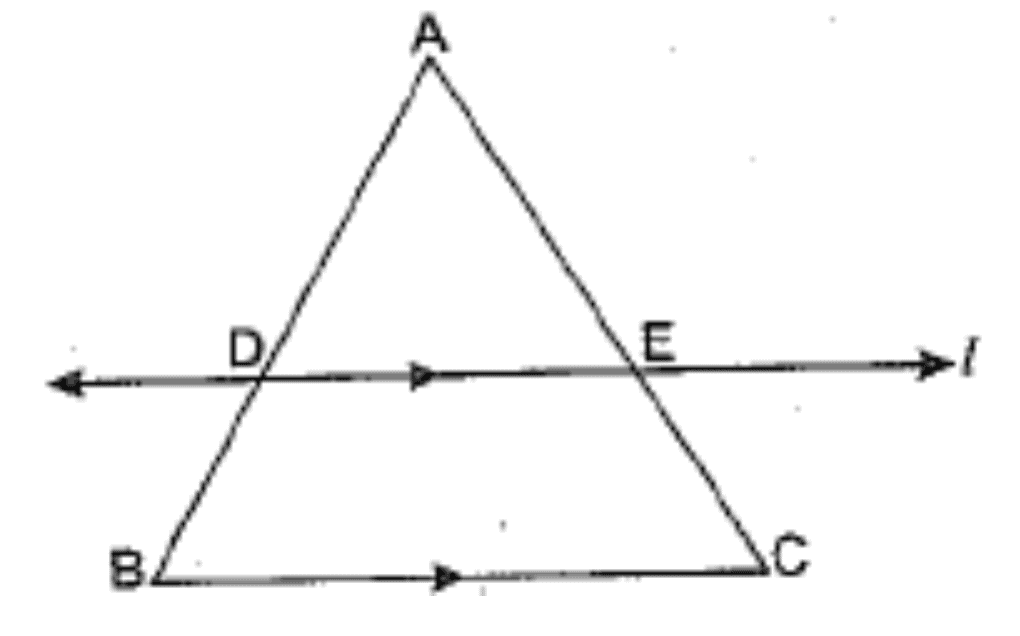Converse of Basic Proportionality Theorem

If a line divides any two sides of a triangle in the same ratio, the line is parallel to the third side i.e., In ΔABC, if l intersects AB in D and AC in E, such that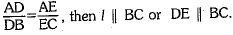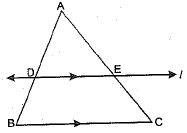Criteria for Similarity of Triangles:

Two triangles are said to be similar, if
(i) their corresponding angles are equal and
(ii) their corresponding sides are in proportion (or are in the same ratio).

(i) AA or AAA Similarity Criterion

If two angles of one triangle are equal to two corresponding angles of another triangle, then the triangles are similar.
If two angles of one triangle are respectively equal to the two angles of another triangle, then the third angles of the two triangles are necessarily equal, because the sum of three angles of a triangle is always 180°.

(ii) SAS Similarity Criterion

If one angle of a triangle is equal to one angle of another triangle and the sides including these angles are in the same ratio, then the two triangles are similar.
Or
If two sides of a triangle are proportional to two corresponding sides of another triangle and the angles included between them are equal, then the triangles are similar.

(iii) SSS Similarity Criterion

If in two triangles, sides of one triangle are proportional (or are in the same ratio) to the sides of the other triangle, then the triangles are similar.
⇒ If ΔABC ~ ΔPQR by any similarity criterion, then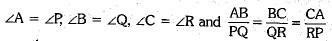i.e., A and P, B and Q, C and R are the corresponding vertices, also AB and PQ, BC and QR, CA and RP are the corresponding sides.

Areas of Similar Triangles

The ratio of the areas of two similar triangles is equal to the square of the ratio of their corresponding sides.
❖ The ratio of the areas of two similar triangles is equal to the square of the ratio of their corresponding medians.
❖ The ratio of the areas of two similar triangles is equal to the ratio of the squares of their corresponding altitudes.
❖ The ratio of the areas of two similar triangles is equal to the ratio of the squares of their corresponding angle bisectors.

Pythagoras Theorem

In a right triangle, the square of the hypotenuse is equal to the sum of the squares of the other two sides.

Converse of Pythagoras Theorem

In a triangle, if the square of one side is equal to the sum of the squares of the other two sides, then the angle opposite the first side is a right angle.
If a perpendicular is drawn from the vertex of the right angle of a right triangle to the hypotenuse, the triangles on each side of the perpendicular are similar to the whole triangle and similar to each other.
i.e., If in ΔABC, ∠B = 90° and BD ⊥ AC, then
(ii) ΔBDC ∼ ΔABC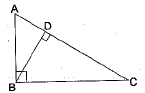RHS Similarity Criterion

If in two right triangles, hypotenuse and one side of one triangle are proportional to the hypotenuse and one side of the other triangle, then the two triangles are similar by RHS similarity criterion.

The document Important Definitions & Formulas: Triangles - Notes | Study Mathematics (Maths) Class 10 - Class 10 is a part of the Class 10 Course Mathematics (Maths) Class 10.
All you need of Class 10 at this link: Class 10

## Mathematics (Maths) Class 10

53 videos|403 docs|138 tests
 Use Code STAYHOME200 and get INR 200 additional OFF

## Mathematics (Maths) Class 10

53 videos|403 docs|138 tests

Track your progress, build streaks, highlight & save important lessons and more!

,

,

,

,

,

,

,

,

,

,

,

,

,

,

,

,

,

,

,

,

,

;